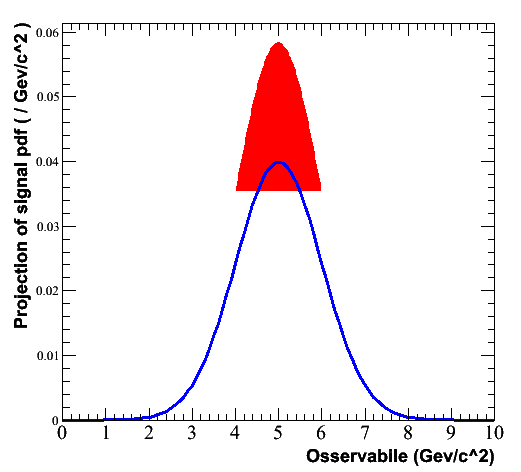# Plot range of PDF

Hi RooFiters,

this is probably a simple question, but I couldn’t find the answer in the forum.

I would like to draw the region of a pdf into a given range over the pdf itself. This is the code I tried:

``````  RooRealVar mass("mass","Observable",0.,10.,"Gev/c^2");
RooRealVar mean("mean","B^0 mass",5.,0.,10.,"Gev/c^2");
RooRealVar width("width","B^0 mass width",1.,0.,3.,"Gev/c^2");

RooGaussian model("model","signal pdf",mass, mean, width);

mass.setRange("signal",4.,6.);

RooPlot *frame = mass.frame();
model.plotOn(frame, Range("signal"), DrawOption("F"),FillColor(kRed));
model.plotOn(frame, Range("Full") );
frame->Draw();``````

and this is what I get: simplestTest.gif

Any hint?

MaurizioHi,

This line will do what you intended to do:

model.plotOn(frame, Range(“signal”,kFALSE), DrawOption(“F”),FillColor(kRed),VLines());

By default Range() sets both the plot range and the normalization range
(which ‘lifts’ the curve in your example). By setting the second argument to kFALSE, you instruct plotOn() to keep the original normalization ranges (which is the full plot Range())

The second modification (VLines()) adds vertical lines downward at the end points of the curve, so that the filled curves filles all the way to zero.
(This reflects that a RooPlot contains TGraphs, which is a lower level
graphics object than e.g. a TH1)

Wouter

Thank you, this is exactly what I needed.

Maurizio